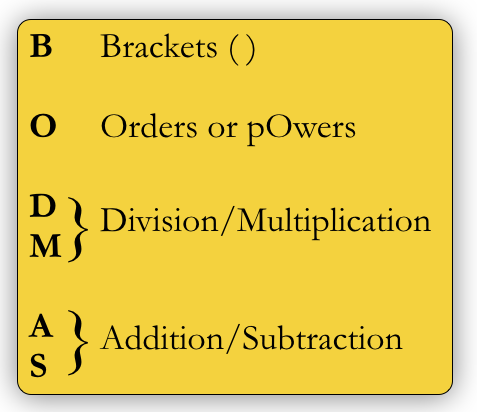# Order of evaluation

Right at the start we talked about the importance of knowing the order of evaluation of operators in an expression and used the standard BODMAS order:Standard order of evaluation

When it comes to

we do the innermost first, that is, the most deeply nested and furthest to the right. This is why when we have an expression like (λy. +  y 1) (+ 2 3) we evaluate the ( + 2 3) part first, if we can. Suppose we have a function (λx. x x), which takes a single parameter and applies it to itself; let’s call that function F. If we try to evaluate (F F), here’s what happens: ((λx. x x) (λx. x x)). We can’t evaluate the one on the right as it is missing parameters, so now we go to the one on the left, and when we apply our $$\beta$$ reduction, we will replace the underlined x variables:

• ((λx. x x) (λx. x x))
• $$\overrightarrow\beta$$ ((λx. x x) (λx. x x))

What just happened here? Each of the two underlined variables was replaced by (λx. x x)so we have the same thing we started with, ((λx. x x) (λx. x x)), and then we repeat the process, and again, and again, for ever.

To see this happen in Racket, use the following function

> (define F (lambda (x) (x x)))
> (F F)


Now imagine we have a function (λx. 3) that takes one parameter and always returns 3, no matter what the value of the parameter is. If we call it with (F F), then we’re stuck with infinite calls because the argument will get evaluated first, but if we don’t evaluate it, we just return the value 3.

The order of evaluation we have been using all along is known as Applicative Order or Eager Evaluation. It works from left to right when evaluating an expression, so, with (λab. * a b) (+ 2 3) (* 1 2), we would start with  (λab. * a b)which immediately tells us that it needs two parameters, to then we work from left to right to evaluate those, giving us:

(λab. * a b) 5 (* 1 2)

Next we do the (* 1 2) to get (λab. * a b) 5 2, and now we can bring in our values for and b.

An alternative method is Normal Order, or the rather delightfully named Lazy Evaluation. Lazy Evaluation delays the evaluation of arguments as long as possible, with a view to not having to evaluate them if they’re not needed. Remember our ((λx. 3) (F F)) example? If we weren’t forced (unnecessarily, as it turns out, because the function doesn’t use it) to evaluate the argument (F F) we could safely evaluate the function. Lazy Evaluation reduces the leftmost outermost redex whenever possible, that is, it works with the outermost level of brackets when it can. This is very different from what you’re likely to have seen before, as typically we go after the most deeply nested brackets.

Here’s an example, consider the expression (λq. * q 2) (* 4 5). Typically we would do a $$\delta$$ conversion on (* 4 5), but with Lazy Evaluation we jump straight to the $$\beta$$ conversion to give us (* (* 4 5) 2). At this point, we can’t work at the outer level so we jump to the innermost and work our way back out as normal, giving us the answer of 40, which is what we would have obtained with Eager Evaluation too. The reason we know to do this is that is a strict function, meaning that all of its arguments must be numbers. This is true of virtually all built in functions like this, so it is a safe bet that if you’re dealing with a function that you didn’t construct yourself, it is a strict function.

What happens when we evaluate ((λx. 3) (F F)) using Lazy Evaluation? We bring in (F F) unchanged, realise that we don’t need it and then throw it way, returning the value 3, which is correct, so Lazy Evaluation is safer. Alas, it’s not all sunshine and puppies; let’s run through this example, first with Eager Evaluation, then with Lazy. It is a lambda calculus function for squaring a number; it takes one parameter and multiplies it by itself:

(λx. * x x)  (* 3 2)

Eager Evaluation will do a $$\delta$$ conversion on (* 3 2) to give:

(λx. * x x)  6

Next we do a  $$\beta$$ conversion giving (* 6 6) and then one more $$\delta$$ conversion for the final answer of 36. That’s three steps.

With Lazy Evaluation, we don’t evaluate (* 3 2) and instead perform a  $$\beta$$ conversion, replacing both instances of x with (* 3 2):

(* (* 3 2) (* 3 2))

Because * is a strict function, we evaluate each argument:

• $$\overrightarrow\delta$$ (* 6 (* 3 2))
• $$\overrightarrow\delta$$ (* 6 6)
• $$\overrightarrow\delta$$ 36

This gives a total of four steps; one  $$\beta$$ conversion and three $$\delta$$ conversions, two of which were the same calculation!

Where does this leave us? There’s no clear winner; Eager Evaluation/Applicative Order can cause infinite calls and can evaluate arguments needlessly, but it evaluates each argument exactly once. Lazy Evaluation/Normal Order, on the other hand, only evaluates arguments when they’re actually being used, but evaluates each argument zero or more times, meaning that it might be more inefficient.

The dream, of course, is to have a situation where we evaluate each argument zero or one times. In that case, we would only evaluate them if we actually need them, but once we do, we would keep track of the result in case we needed it again. This is known as Fully Lazy Evaluation and it is possible, but is outside the scope of this course.